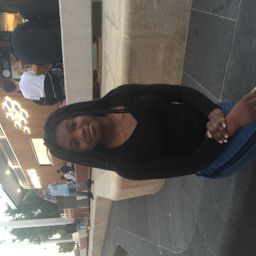Composition

### Discussion

You must be signed in to discuss.
##### Top Chemistry 101 Educators##### Stephanie C.

University of Central Florida##### Allea C.

University of Maryland - University College

### Video Transcript

off it. So here we're solving for an empirical formula of this blue vitriol copper company. So let's line up all of the only once that we have present, we have four copper, sulfur, hydrogen oxygen. Okay, so we've been given a mass percent. Just very hopeful. This is what's gonna go in this column. So over here for copper. They've told us, um, this compound is 25.4% copper, 12.84% sulfur. Um, it's four 0.36% hydrogen and rest is oxygen. Whoa. Comes up to being 57. Who's up? 57.67 percent oxygen. Okay, so these air mass percents, so is our masked values. So we can turn these masses in two moms which will help us find you know, how many out of each Adam is present. We always want to use moles to find that. And you know that most of prevalent to mass overwhelm Asness. So we will take this mass Well, you and divided by the molar mass of the element. So that gives us use quickly. Easier calculator. I already did. It will give us 4.0 four malls, copper first offer. It's also four points your assume. So I took. Let me explain that again if it's too fast. So I took this mass value and it divided it by the molar mass of the element, the molar mass of copper 65 or 63.546 Toy took this mask. Final goodbyes to 63 to get this number for salts for I said 12.4. I divided it by the Mona Lisa sulphur which 32.66 which gives me 0.400 for which is the same thing. Meaning for every copper. I don't have the exact amount of sulfur homes. Now, hydrogen, you take four divided by one. Basically for is, you know, the molar mass. These Hamad glass of hydrogen is around one. It's around 1.8 and 362 divided by four. Excuse me for so now we take this number 57.6 different in divided by a small mass of oxygen. She's 16. It gives us 3.6. Sorry if you can hear that. So, no, What we're gonna do is we're going to find our smallest mole amount Just point for their zero for I'm going toe divi it by each. You know, each of these most take 0.44 divided by is your Are you gonna take 0.4004 divided by itself, which is one. And then we'll take this number divided by itself, which is one again emerging four and divided by this number which will give us 10 as you can, you know, seen. And I'll point for marketing 3.6 divided by this number. And that will give us nine. Yeah, there we have it. So, copper, we have copper. See? So for hydrogen Tene Adams of you quite a lot. And mine. Adams. Hey, oxygen. So I don't know, maybe if you've worked in the lab about your school, you might be familiar with what blue vigil is. Which is copper softly. Penta hydrate. So, um, this is copper stall faint. And then when it's like hydrated with water, we get Penta hydrate and I gives the the bright blue color. It was the very pretty pumped. But this is correct. This is just you know how it's written in real lifeMcMaster University

#### Topics

Composition

##### Top Chemistry 101 Educators##### Stephanie C.

University of Central Florida##### Allea C.

University of Maryland - University College